# What is DFT + embedded DMFT Functional (eDMFT)

eDMFT is a software package implementing the combination Density Functional Theory  and Dynamical Mean Field Theory , which is derived from the stationary Luttinger-Ward functional . The implementation is carefully designed so that it keeps the stationarity of the original functional throughout the algorithm, and postulates the locality of the correlations in real space , as opposed to more commonly chosen locality of correlations in Wannier space. The single-particle Green’s function is expanded in the full potential LAPW basis, and the single-particle Green’s function is self-consistently determined (including self-consistent electronic charge). The algorithm also implements the exact double-counting of DFT and DMFT . The impurity solvers include numerically exact continuous time quantum Monte Carlo , non-crossing and one-crossing approximation .

Current features and capabilities of eDMFT are listed below:

• total free energy and forces in the presence of strong correlations
• exact double-counting of DMFT and LDA
• non-collinear magnetism
• theoretical spectroscopies, such as optics, resistivity, neutron structure factor, resonant elastic X-ray scattering
• several solvers: continuous time quantum Monte Carlo, NCA, OCA
• extreme parallelism (scales up to 100,000 cores) using Message Passing Interface (MPI)
• parallel execution by OpenMP

eDMFT is a flavor of so called DFT+DMFT method, however, in literature DFT+DMFT usually referes to an algorithm whereby DFT is used to determine tight-binding model with the corresponding Wannier orbitals, which are then used to solve a generalized Hubbard model (or sometimes Anderson type lattice model) within DMFT.

DFT+DMFT was first introduced using such downfolding technique , but was later put on more firm footing by defining the stationary functional which combines the two approximations . eDMFT implements one variant of such functional, which is described below.

The existence of such stationary functional is important for stable implementation of the algorithm, because only if the functional exists and is stationary, the implementation is robust, and small error in practical computation leads to negligible error in the final result. For example, in DFT the total energy has second order error in prime variable of interest, the density. Similarly, here the free energy has second order error in the local and static component of the Green’s function.

Notice also that eDMFT implementation does not approximate the itinerant states (or any state) within the tight-binding approximation, rather all valence states are described in the LAPW basis and are allowed to hybridize with the correlated localized subset. The correlated subset, projected to by the projector, is treated dynamically, while the rest of the states are treated at the static mean field level by the DFT, but not removed from the model nor approximated by tight-binding model.

### More precise definition of DFT+eDMFT Functional

#### Luttinger-Ward functional

To compare and combine methods, it is useful to cast them in the same functional language, and perhaps the best choice is the Luttinger-Ward (LW) functional of the form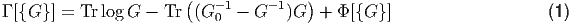where G0(r,r)-1 = δ(r - r)(ω + μ + 2 - V nuclei(r)). The functional is stationary at the exact Green’s function G (δΓ∕δG = 0) as long as the self-energy is computed by Σ = δΦ[{G}]∕δG, because the Dyson equation (G-1 = G 0-1 - Σ) is always enforced. At the stationary point the functional Γ gives the free energy of the system. Within LW approach, approximations are derived by approximating the Φ[{G}] functional, rather than Γ[{G}], because in this case the approximations conserve certain physical quantities, like particle number and energy .

#### DFT within Luttinger-Ward approach

Within the density functional theory (DFT), the prime variable of interest is the electron density ρ(r). The DFT theory in the Luttinger-Ward language is obtained by approximating Φ[{G}] by the Hartree and exchange correlation energy functional, i.e., Φ[{G}] = EH[ρ] + Exc[ρ]. The extremization of the LW functional leads to the well known DFT equations (G-1 = G 0-1 - V H - V xc), which map the many-electron problem onto a single-electron problem in an effective potential (V KS = V H + V xc). Although the exact DFT gives exact total energy and therefore exact value of the free energy Γ at zero temperature, DFT in the Luttinger-Ward approach appears as an approximation, which delivers an approximation for the single-particle Green’s function, and therefore DFT band-structure is seen as the approximation to the true excitation spectra.

Within local density approximation (LDA), the form of the exchange-correlation functional is purely local in 3D space, given by Exc[{ρ}] = d3(r)ε xc[ρ(r)], where εxc(n) is a function of density, determined from the auxiliary system, namely the electron gas. The correlation potential within LDA is then a simple function of εxc(n), and is given by V xc = δExc∕δρ = εxc + εxcρ. We hence conclude that in order to determine the correlation potential within LDA, each point in space is mapped to an auxiliary problem of electron gas, and each point in space is treated as independent in this step. It is due to the kinetic energy, the Hartree part of the Hamiltonian and the Dyson equation that different points in space get coupled, and the band structure with momentum dependence appears. As the DFT band structures in most weakly correlated materials are reasonable, we can conclude that even purely local approximation on LW functional Φ[{G}] (an approximation in which each point in space is independently mapped to an auxiliary problem) is a reasonable starting point. This extreme locality of the exchange-correlation functional in real materials is the reason for the extraordinary success of approximate DFT functionals such as LDA or GGA.

#### DMFT within Luttinger-Ward approach

DMFT was originally developed  in the context of the Hubbard model considering the limit of large dimensions  (or connectivity), where DMFT becomes exact. The DMFT approximation can also be cast into an extremizing problem of the LW functional, in which Φ[{G}] is approximated by the local functional Φ[{GlocalR}], but in DMFT the locality to the given site on the lattice R is enforced, rather than locality to a given point in 3D space. In addition, the functional form of ΦDMFT is kept the same as the exact Φ[{G}], except that the variable of interest is truncated from the G(r,r) to Glocal(r,r). Namely, Glocal(r,r) is the local component of G and vanishes when r and rare on different atoms. Due to the fact that ΦDMFT is so closely connected to the exact functional, it must be dynamic, and it develops a singularity at sufficiently strong Coulomb interaction, as envisioned by Mott in 1930 . Mathematically, one can write the DMFT functional as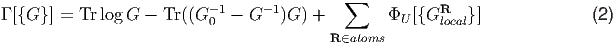where ΦU[{GlocalR}] is the sum of all skeleton Feynman diagrams constructed by the Coulomb interaction Û and GlocalR. The topology and form of these Feynman diagrams is the same as those contained in the exact Φ[{G}].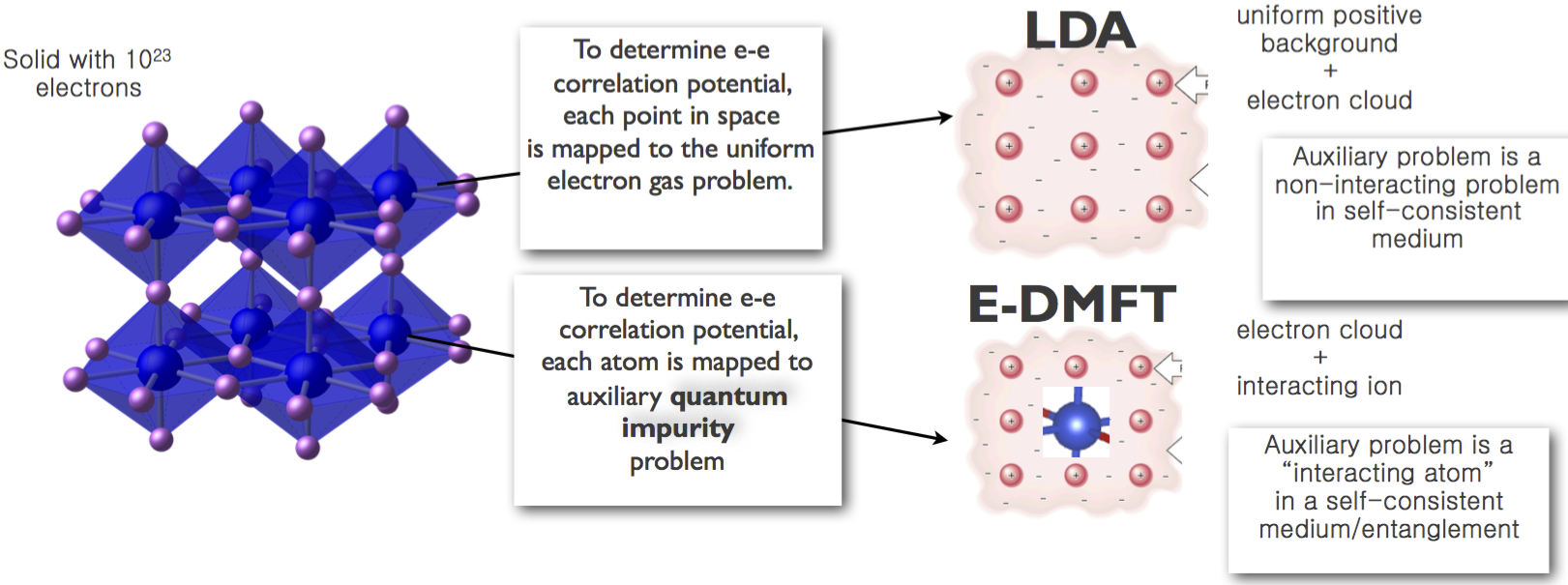Figure 1: A schematic illustration of similarity between the two "local” approximations LDA and DMFT. The locality in the former is to a point in 3D space, while in the latter is to an ion in the solid.

Notice the similarity of the two local approximations, LDA and DMFT, in which the LDA functional can be expressed by ΦLDAXC = d3rΦxc[G(rt,rt)δ(r - r)δ(t-t)] and the DMFT by ΦDMFT XC = RΦxc[G localR]. Both thus truncate the observable of interest in real space, as shown in Fig. 2, but LDA is local to a point in 3D space, while DMFT is local to an atom in the lattice. Notice also that the LDA functional delivers the exact energy and the exact charge density in the limit of uniform density, while DMFT gives the exact solution in the limit of large lattice connectivity, not just the ground state, but also the exact excitations. While the form of the wave function of the DMFT problem is not known, one can nevertheless compute any correlation function of the system, which can be computed with the impurity solver.

#### DFT+eDMFT Functional

Finally, the combination of DFT and eDMFT approximates the Φ[{G}] functional with the combination of DFT and DMFT terms ΦLDA[{G}] + ΦDMFT [{G}] - ΦDC[{G}]. In principle one can define the DMFT-type approximation which would treat on the DMFT level all degrees of freedom , but current impurity solvers can not treat much more than seven orbitals (an open f shell), therefore a good choice is to treat more itinerant degrees of freedom on the DFT level, and add all Feynman diagrams only for those narrow orbitals in which correlations are strongest. Mathematically, the LW functional to be extremized is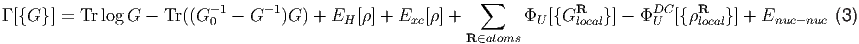where Enuc-nuc is the nuclear contribution to the energy. Because the functional ΦU[{GlocalR}] contains only a single interacting site (or a cluster of sites), one can see that the same sum of diagrams appears also in the solution of an auxiliary quantum impurity problem Φimp[{Gimp}] (quantum cluster problem), which must have the same Green’s function Gimp = Glocal, and the same local Coulomb interaction Û. Hence, ΦU[{GlocalR}] can be computed exactly by solving an auxiliary quantum impurity problem. This can now be achieved numerically exactly by the continuous time quantum Monte Carlo (CTQMC) solver 

In order to fully define the DFT+DMFT approximation, one needs to specify the projector to the local Green’s function, i.e., GlocalR =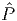RG. Most early DFT+DMFT implementations used the Wannier basis constructed from a minimal set of bands, and mapping the problem to a Hubbard-like model. It was later shown  , that a better choice for DMFT projector is a real space projector, constructed by set of quasiatomic orbitals, which are much more localized than the Wannier orbitals, and for which the negligence of intersite correlations is much less severe. This is because correlations in real space are very local, but they are not necessary local in Wannier basis.

In order to preserve stationarity of the DFT+DMFT functional, the projector must be independent of the self-consistent charge density, therefore it needs to be fixed during the DMFT optimization. We recommend the following choice for the projector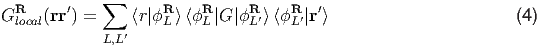where ϕLR is a set of basis functions centered on atom at R, and the best choice for ϕLR are the solutions of the Dirac equation (at the LDA level) inside the muffin-tin sphere at the energy corresponding to the Fermi energy. (This is projector 5 in the code.)

Notice that in such formulation of DFT+DMFT, all valence states are kept in the model and are allowed to hybridize with the correlated localized subset. The correlated subset, projected to by the projector, is treated dynamically, while the rest of the states are treated at the static mean field level by the DFT, but not removed from the model. Moreover, the screened Coulomb repulsion Û among the electrons in such a real space projectors is larger and more universal among similar classes of materials .

Exact double-counting : The double-counting problem arises from the fact that DFT (in its approximations such as LDA or GGA) and the DMFT, each contain some form of correlations, but what exactly is contained in both approximations was considered unknown. Several simplistic approximations derived in the context of LDA+U, and obtained in certain limits such as localized limit or mean-field limit, were used in practice.

In Refs.  the exact overlap between the dynamical mean field theory and the band structure methods was derived quite generally using the Luttinger-Ward functional language introduced above. The basic idea is that ΦDMFT and ΦLDA in Eq. 3 are two different approximations of the same exact LW functional Φexact, and we know exactly how to get from Φexact to ΦDMFT or to ΦLDA. To obtain the LDA approximation, we truncate in Φexact the Green’s function to its static and local part, i.e., ρ(r) = G(rr)δ(r - r)δ(τ - τ), hence the charge is the observable, and then map each point in space to the problem of electron gas. To get the DMFT approximation, we truncate in Φexact the Green’s function to the following local component Gloc(r,r) =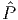G(r,r) = α,β r|ϕαϕα|G|ϕβϕβ|r, and also replace the bare Coulomb repulsion vC(r,r) =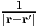with the screened Coulomb repulsion vDMFT (r,r) ( which obeys Uαβγδ = ϕαϕβ|vDMFT |ϕγϕδ). To obtain the overlap between the two approximations, one can apply first the LDA approximation on the exact functional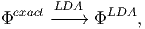(5)

and then apply the DMFT approximation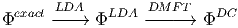(6)

to obtain the double-counted correlations. Alternatively, one can first start with DMFT approximation and then apply the LDA approximation to it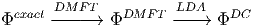(7)

and also get the same functional. The double-counted functional can be written asand is a variable of local density ρlocal(r) = δ(τ - τ) α,β r|ϕαϕα|G|ϕβϕβ|r and the screened Coulomb repulsion vDMFT . To evaluate the exchange-correlation part, one needs to map each point in space to the problem of electron gas, in which electrons interact with the screened Coulomb repulsion vDMFT (r,r). We derived in Ref.  the two dimensional parametrization of such exchange correlation energy εxc[λ,ρ] (where λ is the screening length in yukawa-like screened interaction) which is used in the eDMFT implementation. The tests show that this double-counting is superior to all other approximate forms of DC, and that the nominal-DC, introduced in Ref.  is the best approximation to it. We also found recently that in some insulators, screening is better approximated by a combination of yukawa and dielectric screening, i.e., vDMFT =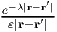, and the derivation of such exchange-correlation energy is available in the eDMFT tutorials  and implemented in the code.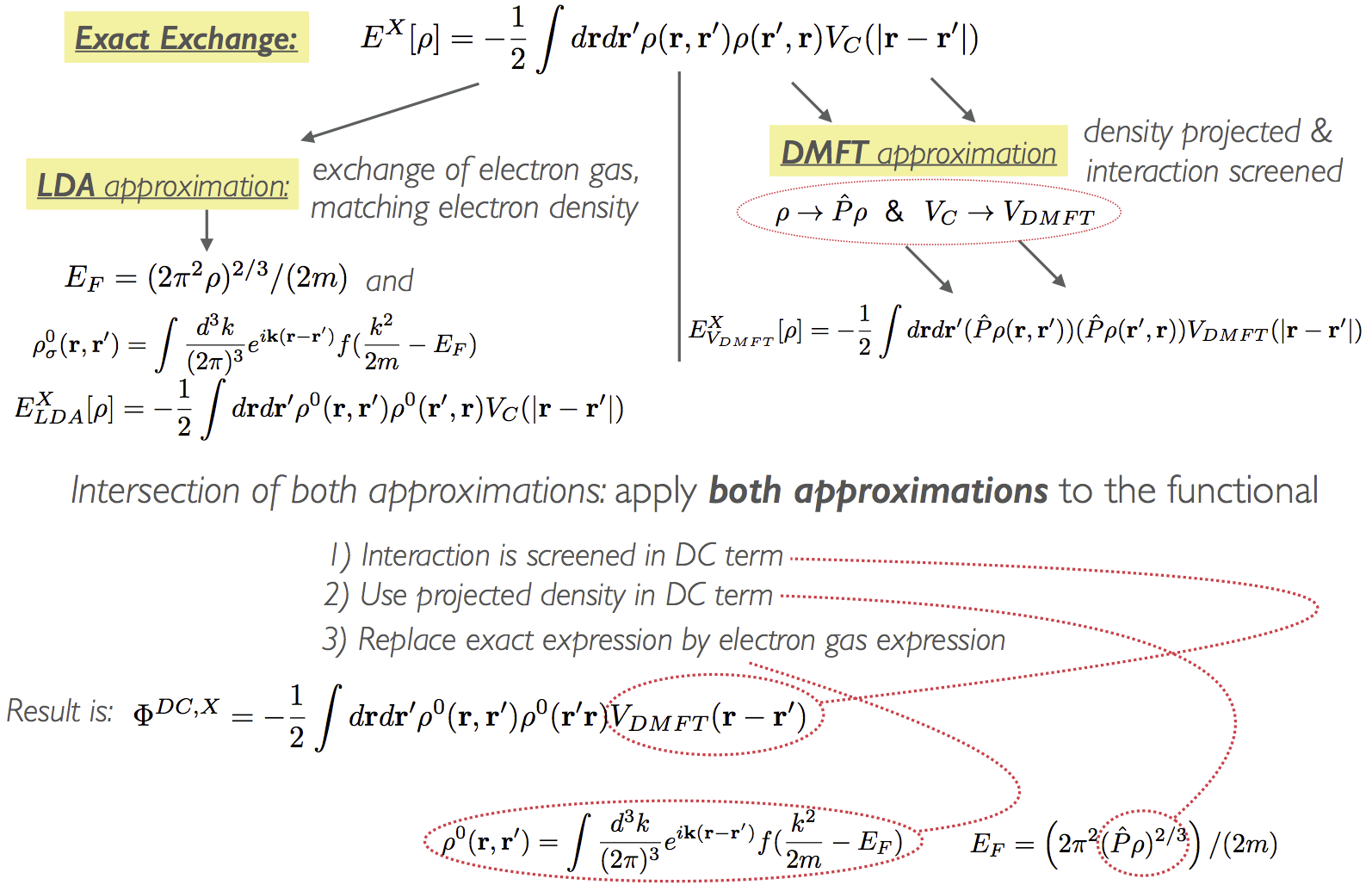Figure 2: The sketch of how to obtain the exact double-counting of the exchange functional within the Luttinger-Ward approach.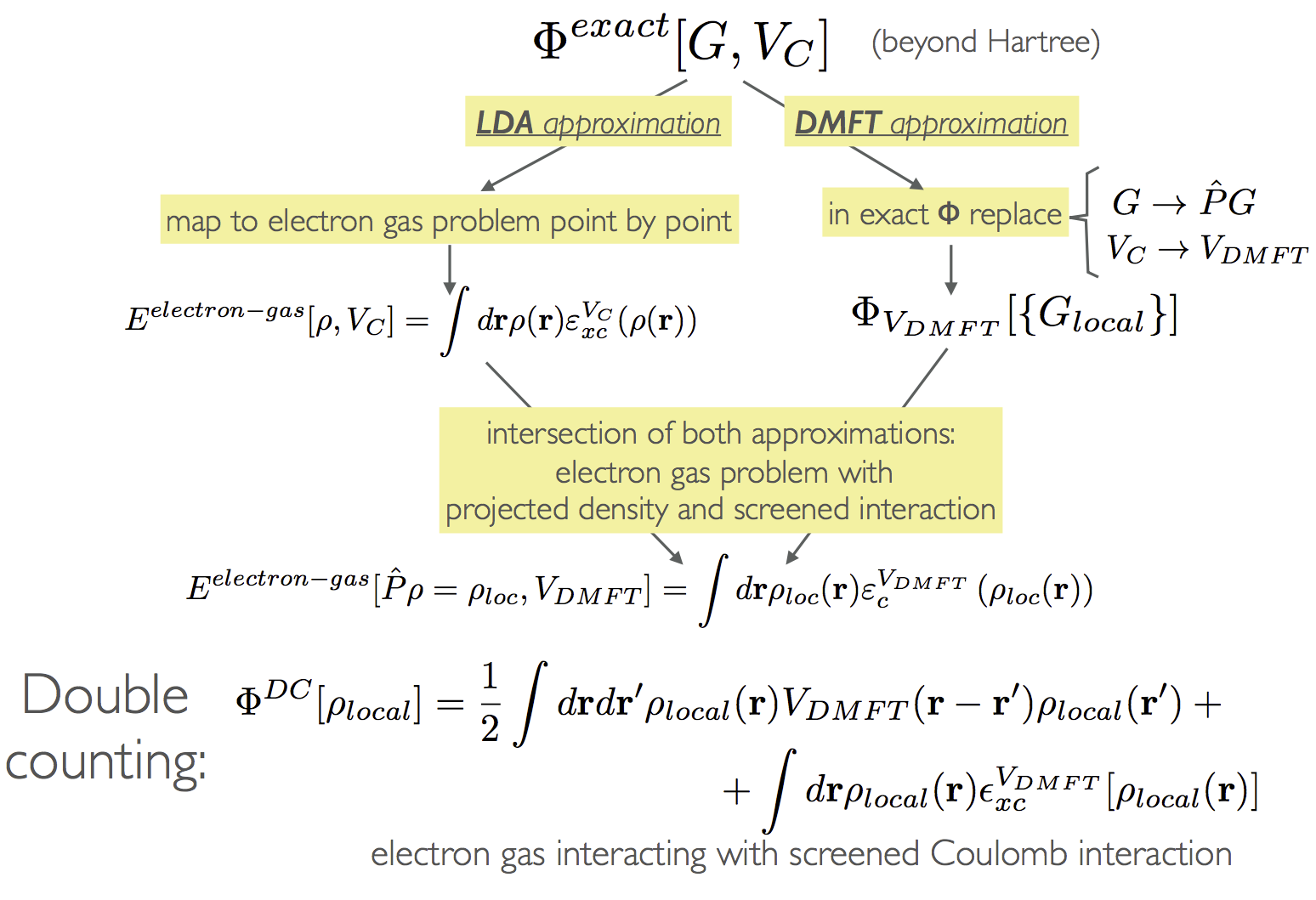Figure 3: The sketch of how to obtain the exact double-counting of the correlation functional.

Total Free Energy and Forces : One possible way to compute the total energy of the system, is to use so-called Migdal-Galitskii formula to compute the DMFT potential energy (EDMFT M-Galitskii =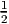TrG)) which can then be added to the LDA/GGA total energy. The total energy expression in this approach reads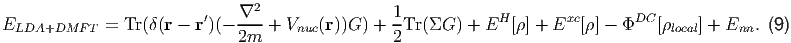It turns out (see Ref. ) that this expression for the energy is not stationary because lim T0FLDA+DMFT = ELDA+DMFT M-Galitskii does not hold in the charge self-consistent DFT+DMFT, governed by the functional Eq. 3. This is because Gordon Baym’s proof  for conserving approximations does not apply to self-consistent DFT+DMFT, as LDA/GGA is not a conserving approximations in Baym’s sense, as it clearly does not obey the relationship Exc12Tr(V xcρ).

However, within eDMFT functional approach, the free energy of the system is given by the LW functional: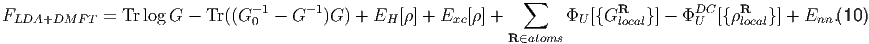This formula was implemented in Ref. , and is available in current eDMFT implementation.

Real usefulness and power of the free energy functional expression is evident for the calculation of forces. The force is defined as the change of the total energy when an atom is displaced F = -δE∕δR. The availability of forces on all atoms is essential for structural relaxations, and for computing phonons. Prior attempt to compute forces within DFT+DMFT was based on differentiating ELDA+DMFT M-Galitskii. The problem is that in this case the force contains a term, which requires one to compute the two-particle vertex function (ΓV = δΣ∕δG), which depends on three frequencies and four orbital indices, which is numerically extremely hard to compute.

The exact force at finite temperature should however be computed by differentiating the free energy expression FLDA+DMFT , which is stationary. The consequence of stationarity is that the vertex function ΓV does not appear in the final expression for the force. More precisely, if the implementation of the force is carried out within a complete and origin-less basis set, such as the plane waves, than the force is given just by the Hellmann-Feynman Force (see Ref. ). Our LAPW basis set contains also the atom-centered basis functions, which generate so-called Pulley forces. In Ref.  this force was implemented, and it was found quite surprisingly that the computation of the force is numerically more stable than computation of the free energy formula Eq. 10. This is because it is numerically quite hard to compute the DMFT Luttinger-Ward functional ΦDMFT , which appears in the free energy. On the other hand, the force expression depends only on its derivative δΦDMFT ∕δG, which is the DMFT self-energy, and can be computed very precisely by the continuous time quantum Monte Carlo method .

### References

    P. Hohenberg and W. Kohn. Inhomogeneous electron gas. Phys. Rev., 136:B864–B871, Nov 1964. doi: 10.1103/PhysRev.136.B864. URL http://link.aps.org/doi/10.1103/PhysRev.136.B864.

    Antoine Georges, Gabriel Kotliar, Werner Krauth, and Marcelo J. Rozenberg. Dynamical mean-field theory of strongly correlated fermion systems and the limit of infinite dimensions. Rev. Mod. Phys., 68:13–125, 1996. URL http://link.aps.org/doi/10.1103/RevModPhys.68.13.

    G. Kotliar, S. Y. Savrasov, K. Haule, V. S. Oudovenko, O. Parcollet, and C. A. Marianetti. Electronic structure calculations with dynamical mean-field theory. Rev. Mod. Phys., 78:865–951, 2006. URL http://link.aps.org/doi/10.1103/RevModPhys.78.865.

    Kristjan Haule and Turan Birol. Free energy from stationary implementation of the DFT + DMFT functional. Phys. Rev. Lett., 115:256402, Dec 2015. doi: 10.1103/PhysRevLett.115.256402. URL http://link.aps.org/doi/10.1103/PhysRevLett.115.256402.

    Kristjan Haule, Chuck-Hou Yee, and Kyoo Kim. Dynamical mean-field theory within the full-potential methods: Electronic structure of CeIrIn5, CeCoIn5, and CeRhIn5. Phys. Rev. B, 81: 195107, 2010. URL http://link.aps.org/doi/10.1103/PhysRevB.81.195107.

    Kristjan Haule. Exact double counting in combining the dynamical mean field theory and the density functional theory. Phys. Rev. Lett., 115:196403, Nov 2015. doi: 10.1103/PhysRevLett.115.196403. URL http://link.aps.org/doi/10.1103/PhysRevLett.115.196403.

    Kristjan Haule. Quantum monte carlo impurity solver for cluster dynamical mean-field theory and electronic structure calculations with adjustable cluster base. Phys. Rev. B, 75:155113, Apr 2007. doi: 10.1103/PhysRevB.75.155113. URL http://link.aps.org/doi/10.1103/PhysRevB.75.155113.

    K. Haule, S. Kirchner, J. Kroha, and P. Wölfle. Anderson impurity model at finite coulomb interaction U : Generalized noncrossing approximation. Phys. Rev. B, 64:155111, Sep 2001. doi: 10.1103/PhysRevB.64.155111. URL http://link.aps.org/doi/10.1103/PhysRevB.64.155111.

    V. I. Anisimov, A. I. Poteryaev, M. A. Korotin, A. O. Anokhin, and G. Kotliar. First-principles calculations of the electronic structure and spectra of strongly correlated systems: dynamical mean-field theory. Journal of Physics: Condensed Matter, 9(35):7359–7367, 1997. URL http://stacks.iop.org/0953-8984/9/i=35/a=010.

    A. I. Lichtenstein and M. I. Katsnelson. Ab initio calculations of quasiparticle band structure in correlated systems: LDA++ approach. Phys. Rev. B, 57: 6884–6895, 1998. URL http://link.aps.org/doi/10.1103/PhysRevB.57.6884.

    Gordon Baym and Leo P. Kadanoff. Conservation laws and correlation functions. Phys. Rev., 124:287–299, Oct 1961. doi: 10.1103/PhysRev.124.287. URL http://link.aps.org/doi/10.1103/PhysRev.124.287.

    Walter Metzner and Dieter Vollhardt. Correlated lattice fermions in d=dimensions. Phys. Rev. Lett., 62:324–327, 1989. URL http://link.aps.org/doi/10.1103/PhysRevLett.62.324.

    N F Mott and R Peierls. Discussion of the paper by de boer and verwey. Proceedings of the Physical Society, 49(4S):72–73, 1937. URL http://stacks.iop.org/0959-5309/49/i=4S/a=308.

    N. F. Mott. Metal Insulator Transition. Taylor & Francis London, 1990. URL http://badmetals.magnet.fsu.edu/pdfs/Mott90book-chap8.pdf.

    S. Y. Savrasov and G. Kotliar. Spectral density functionals for electronic structure calculations. Phys. Rev. B, 69:245101, Jun 2004. doi: 10.1103/PhysRevB.69.245101. URL http://link.aps.org/doi/10.1103/PhysRevB.69.245101.

    P. Sémon, Chuck-Hou Yee, Kristjan Haule, and A.-M. S. Tremblay. Lazy skip-lists: An algorithm for fast hybridization-expansion quantum monte carlo. Phys. Rev. B, 90:075149, Aug 2014. doi: 10.1103/PhysRevB.90.075149. URL http://link.aps.org/doi/10.1103/PhysRevB.90.075149.

    Kristjan Haule, Turan Birol, and Gabriel Kotliar. Covalency in transition-metal oxides within all-electron dynamical mean-field theory. Phys. Rev. B, 90:075136, Aug 2014. doi: 10.1103/PhysRevB.90.075136. URL http://link.aps.org/doi/10.1103/PhysRevB.90.075136.

    Z. P. Yin, K. Haule, and G. Kotliar. Kinetic frustration and the nature of the magnetic and paramagnetic states in iron pnictides and iron chalcogenides. Nat Mater, 10:932, 2011. URL http://dx.doi.org/10.1038/nmat3120.

    Juho Lee and Kristjan Haule. Dynamical mean field theory for diatomic molecules and the exact double counting. Phys. Rev. B, 91:155144, Apr 2015. doi: 10.1103/PhysRevB.91.155144. URL http://link.aps.org/doi/10.1103/PhysRevB.91.155144.

    Gordon Baym. Self-consistent approximations in many-body systems. Phys. Rev., 127:1391–1401, Aug 1962. doi: 10.1103/PhysRev.127.1391. URL http://link.aps.org/doi/10.1103/PhysRev.127.1391.

    Kristjan Haule and Gheorghe L. Pascut. Forces for structural optimizations in correlated materials within dft+embedded dmft functional approach. arXiv:1602.02819, 2016. URL http://lanl.arxiv.org/abs/1602.02819.